Case Based Questions (MCQ)

Chapter 2 Class 10 Polynomials
Serial order wise

## Parabola A parabola is the graph that results from p(x) = ax 2 + bx + c. Parabolas are symmetric about a vertical line known as the Axis of Symmetry. The Axis of Symmetry runs through the maximum or minimum point of the parabola which is called the vertex.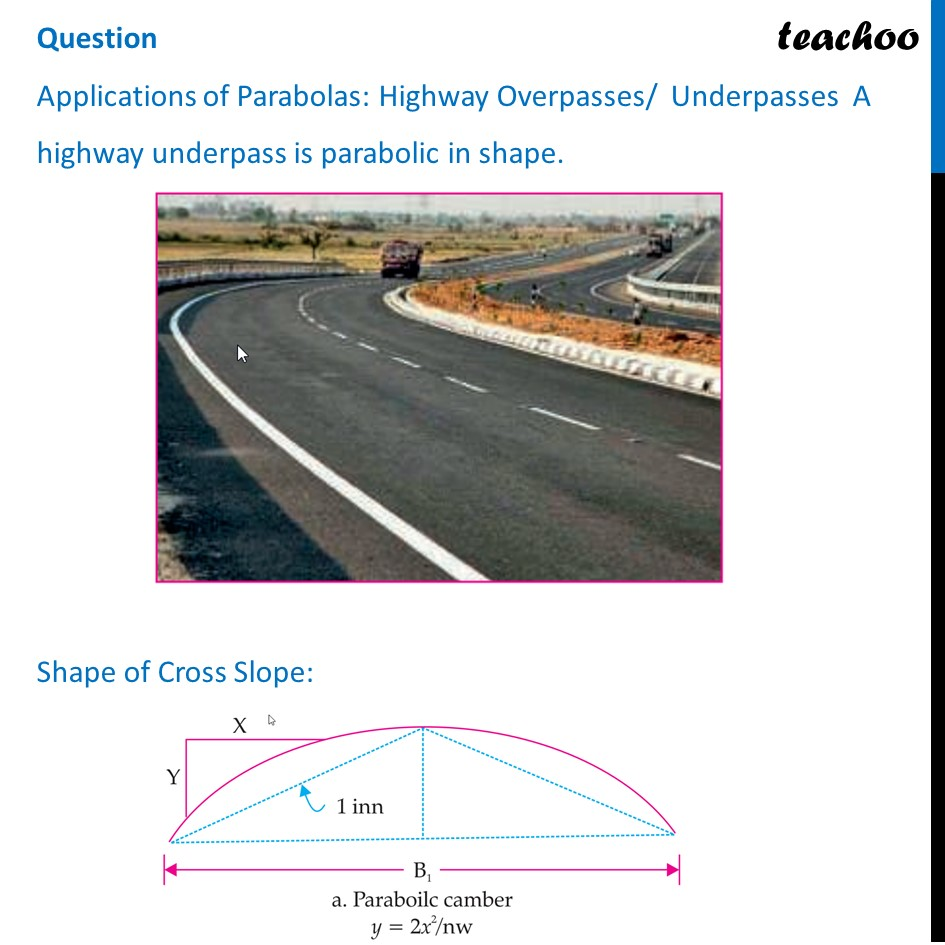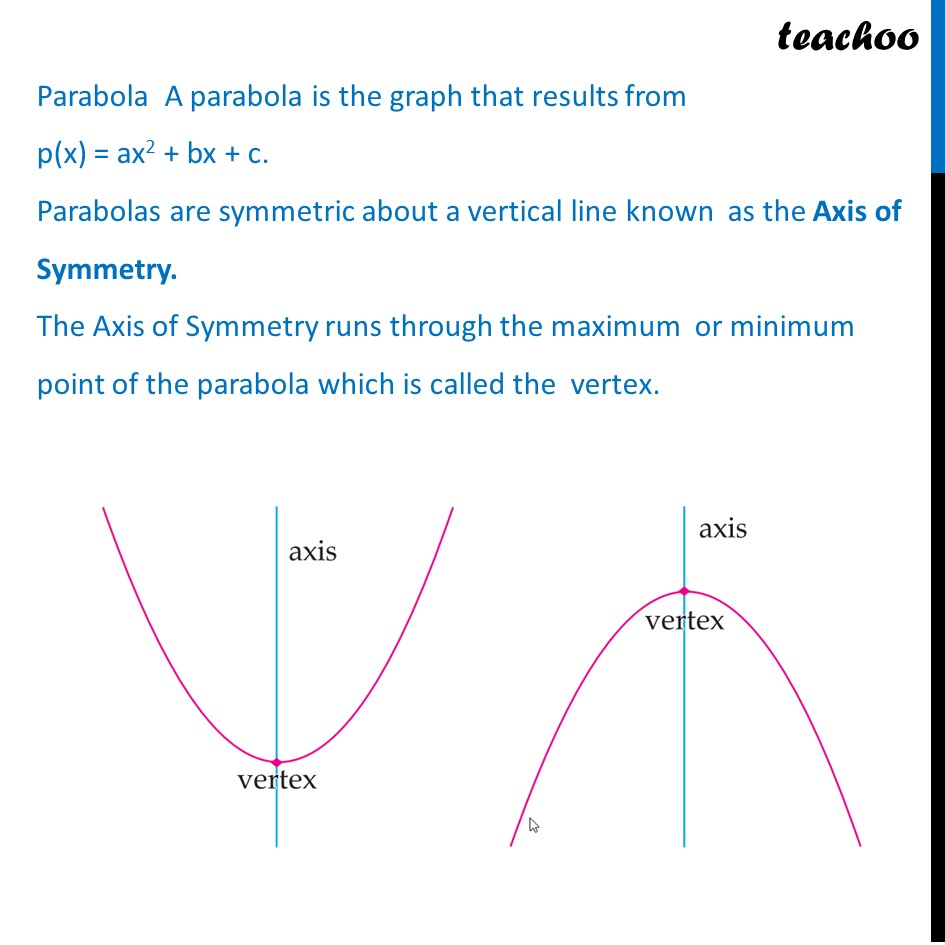## (d) (– 4, – 4)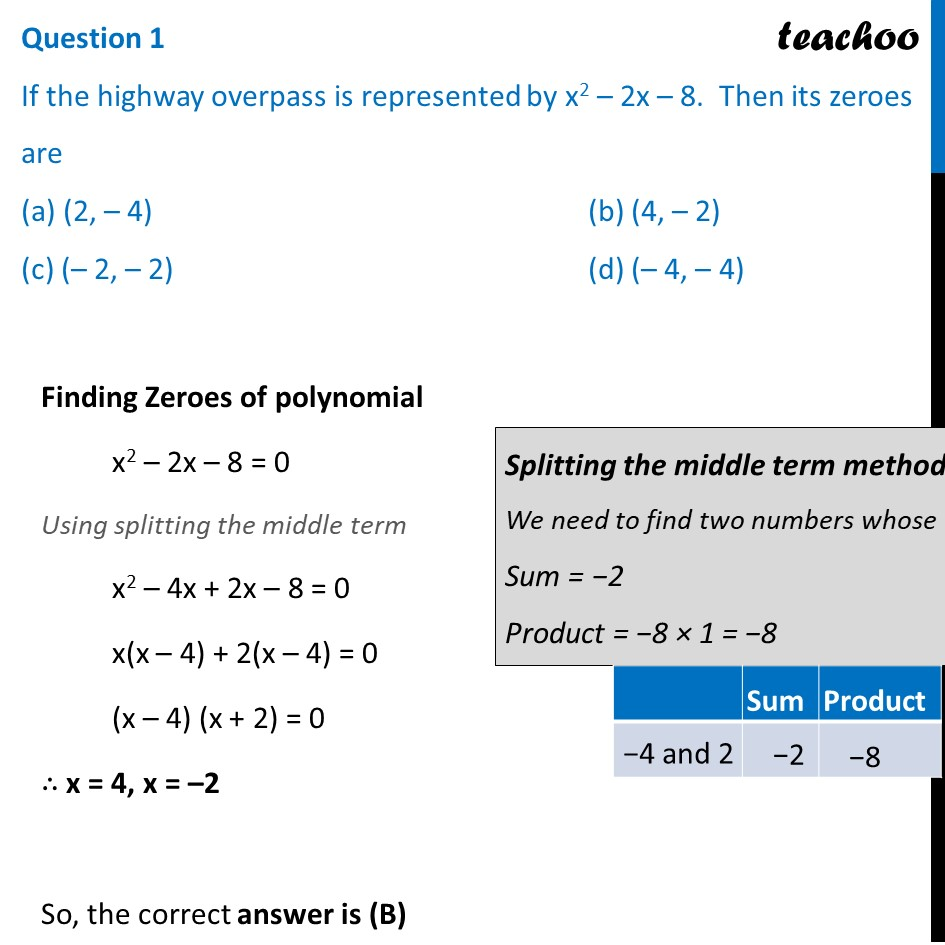## (d) None of the above## (d) ellipse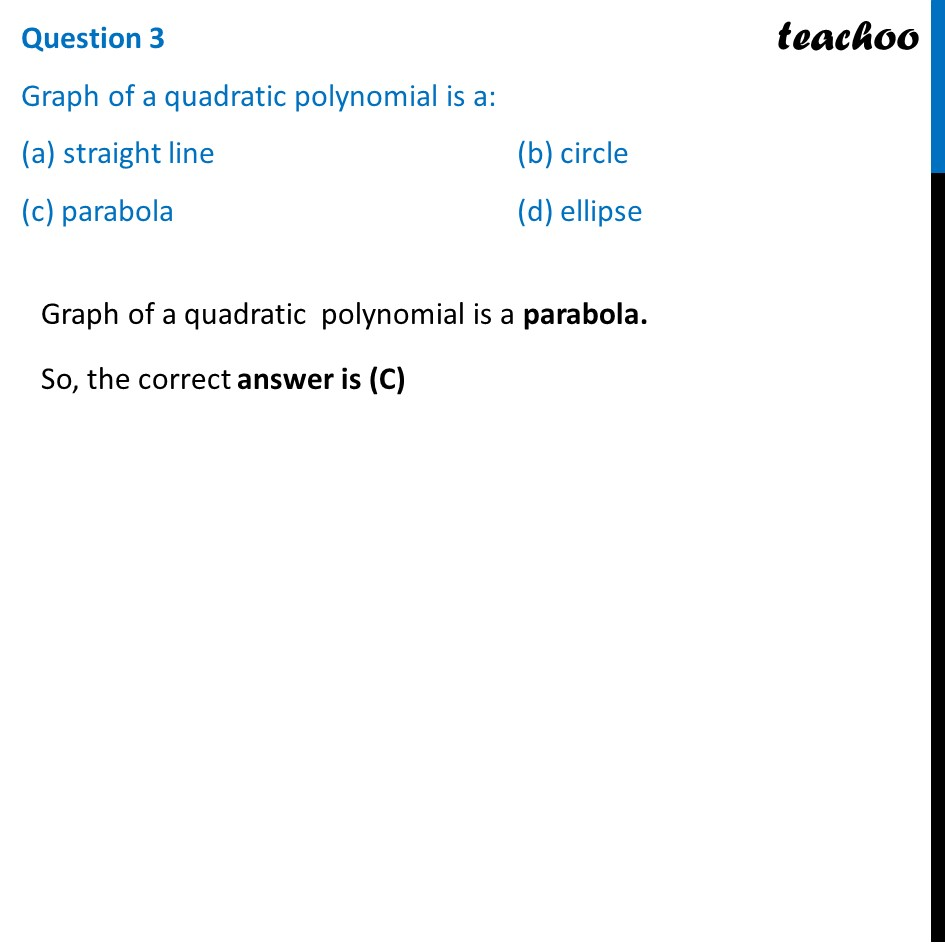## (d) x 2 – 3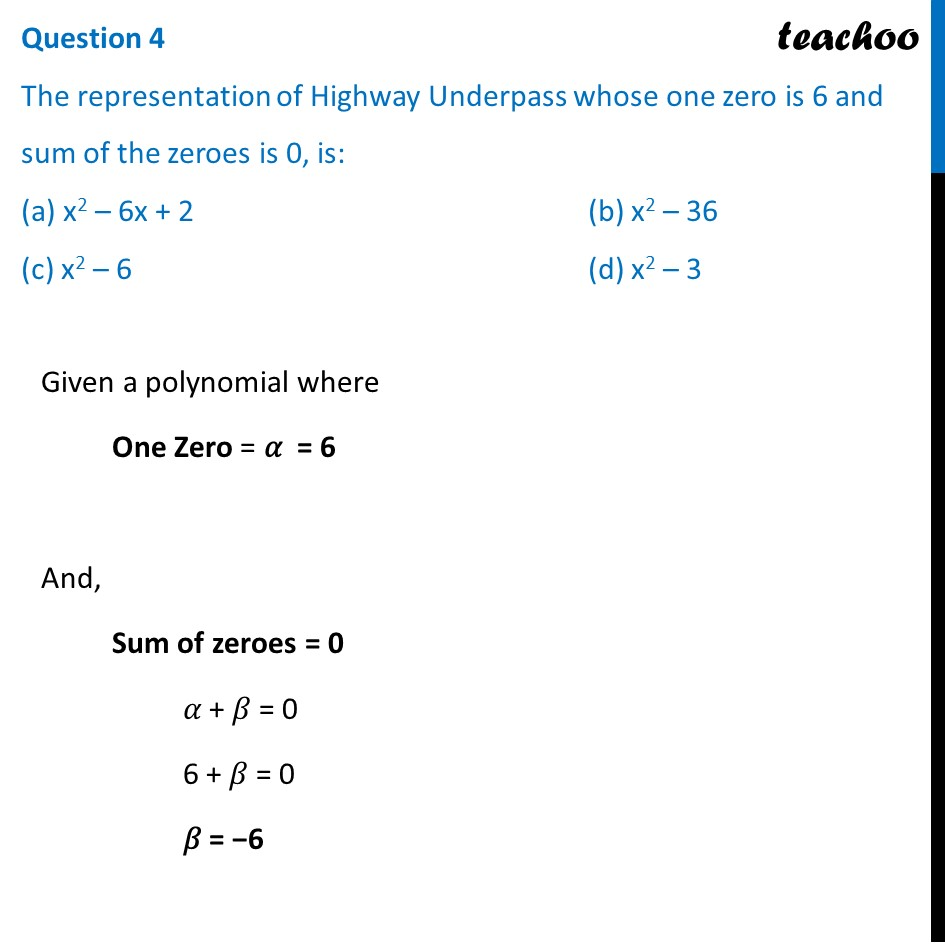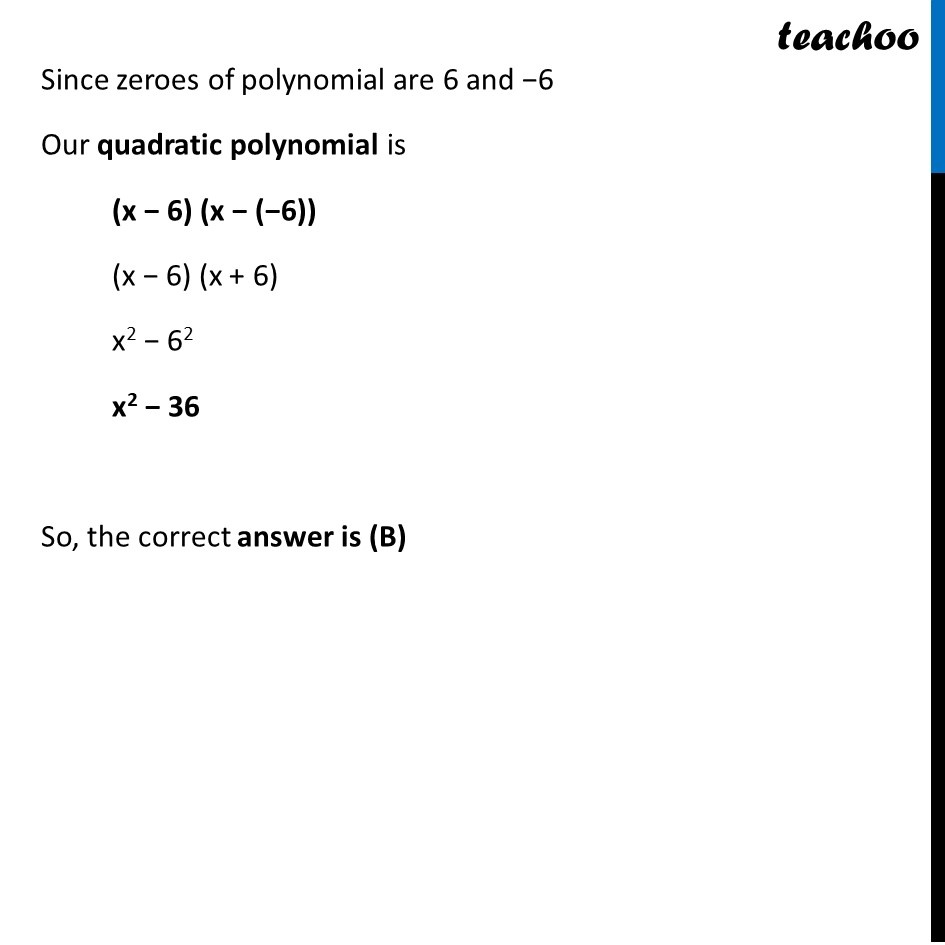## (d) 3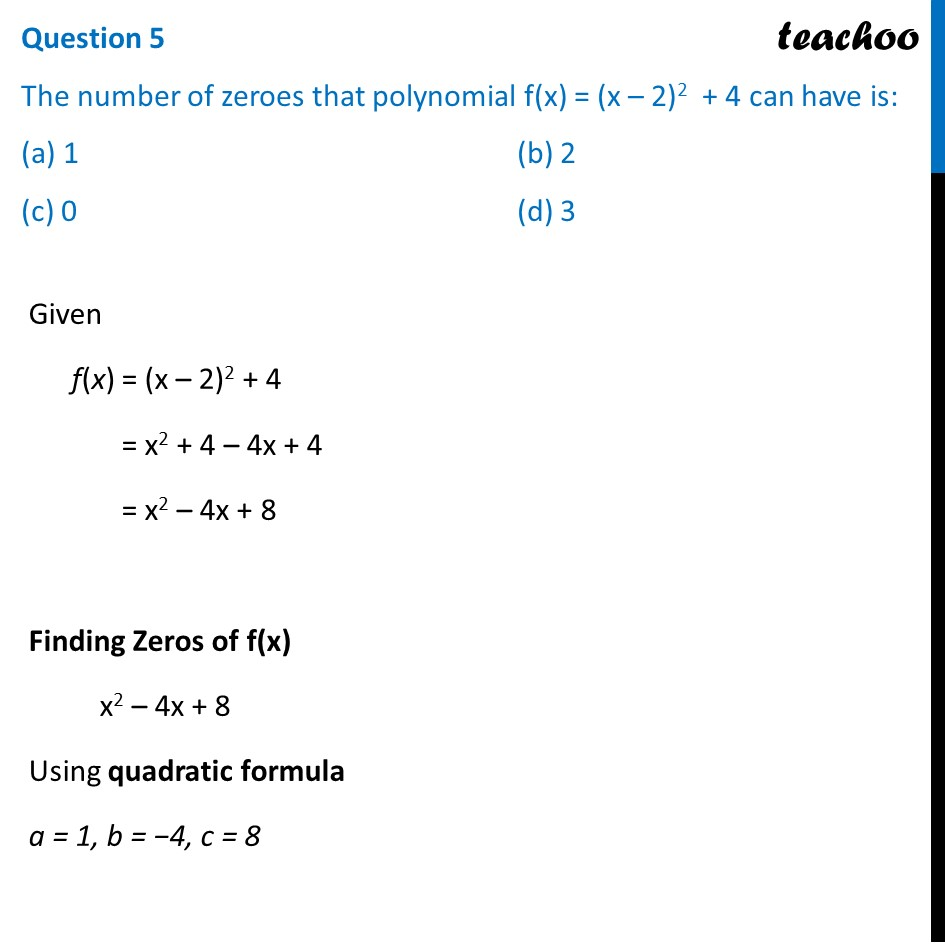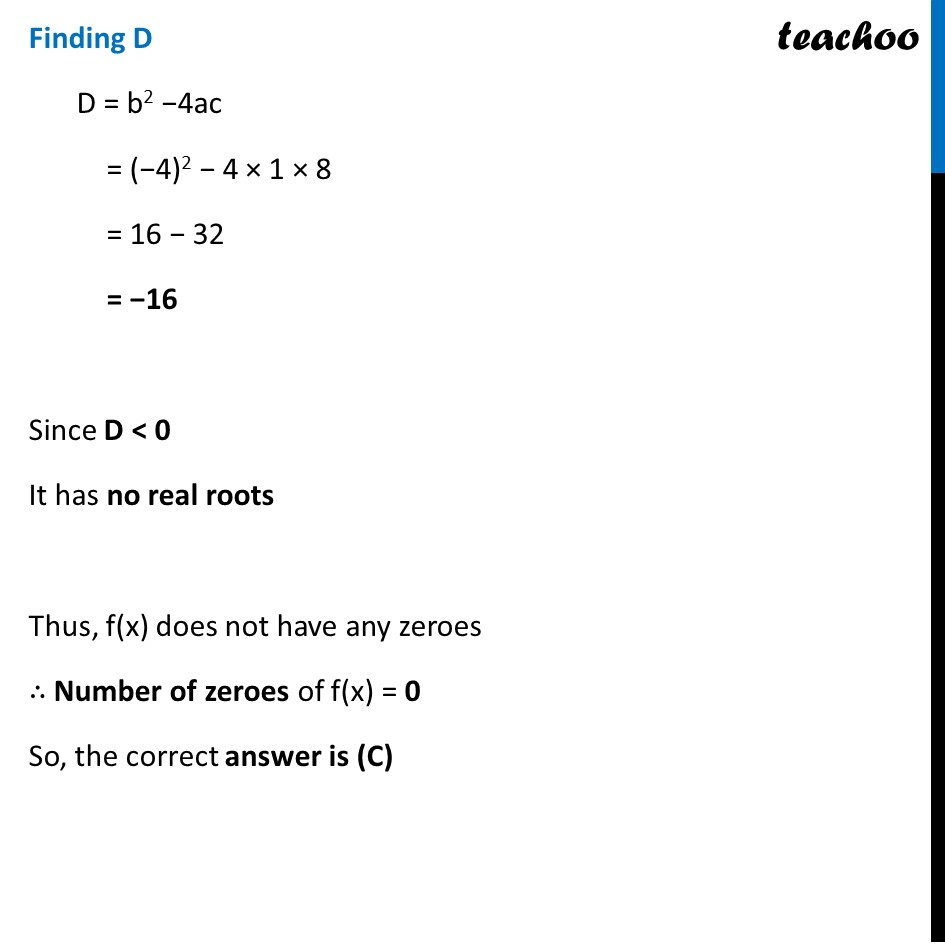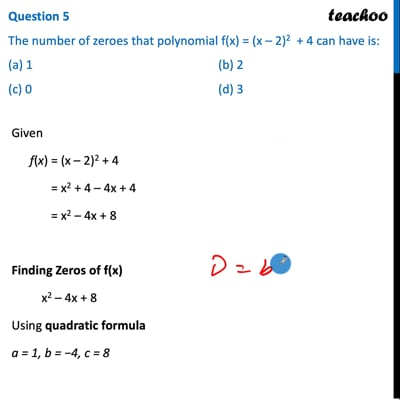This video is only available for Teachoo black users

Introducing your new favourite teacher - Teachoo Black, at only ₹83 per month

### Transcript

Question Applications of Parabolas: Highway Overpasses/ Underpasses A highway underpass is parabolic in shape. Shape of Cross Slope: Parabola A parabola is the graph that results from p(x) = ax2 + bx + c. Parabolas are symmetric about a vertical line known as the Axis of Symmetry. The Axis of Symmetry runs through the maximum or minimum point of the parabola which is called the vertex. Question 1 If the highway overpass is represented by x2 – 2x – 8. Then its zeroes are (a) (2, – 4) (b) (4, – 2) (c) (– 2, – 2) (d) (– 4, – 4) Finding Zeroes of polynomial x2 – 2x – 8 = 0 Using splitting the middle term x2 – 4x + 2x – 8 = 0 x(x – 4) + 2(x – 4) = 0 (x – 4) (x + 2) = 0 ∴ x = 4, x = –2 So, the correct answer is (B) Question 2 The highway overpass is represented graphically. Zeroes of a polynomial can be expressed graphically. Number of zeroes of polynomial is equal to number of points where the graph of polynomial: (a) Intersects X-axis (b) Intersects Y-axis (c) Intersects Y-axis or X-axis (d) None of the above We know that Number of zeroes is equal to number of times parabola intersects the x-axis So, the correct answer is (A) Question 3 Graph of a quadratic polynomial is a: (a) straight line (b) circle (c) parabola (d) ellipse Graph of a quadratic polynomial is a parabola. So, the correct answer is (C) Question 4 The representation of Highway Underpass whose one zero is 6 and sum of the zeroes is 0, is: (a) x2 – 6x + 2 (b) x2 – 36 (c) x2 – 6 (d) x2 – 3 Given a polynomial where One Zero = 𝛼 = 6 And, Sum of zeroes = 0 𝛼 + 𝛽 = 0 6 + 𝛽 = 0 𝛽 = −6 Since zeroes of polynomial are 6 and −6 Our quadratic polynomial is (x − 6) (x − (−6)) (x − 6) (x + 6) x2 − 62 x2 − 36 So, the correct answer is (B) Question 5 The number of zeroes that polynomial f(x) = (x – 2)2 + 4 can have is: (a) 1 (b) 2 (c) 0 (d) 3 Given f(x) = (x – 2)2 + 4 = x2 + 4 – 4x + 4 = x2 – 4x + 8 Finding Zeros of f(x) x2 – 4x + 8 Using quadratic formula a = 1, b = −4, c = 8 Finding D D = b2 −4ac = (−4)2 − 4 × 1 × 8 = 16 − 32 = −16 Since D < 0 It has no real roots Thus, f(x) does not have any zeroes ∴ Number of zeroes of f(x) = 0 So, the correct answer is (C)